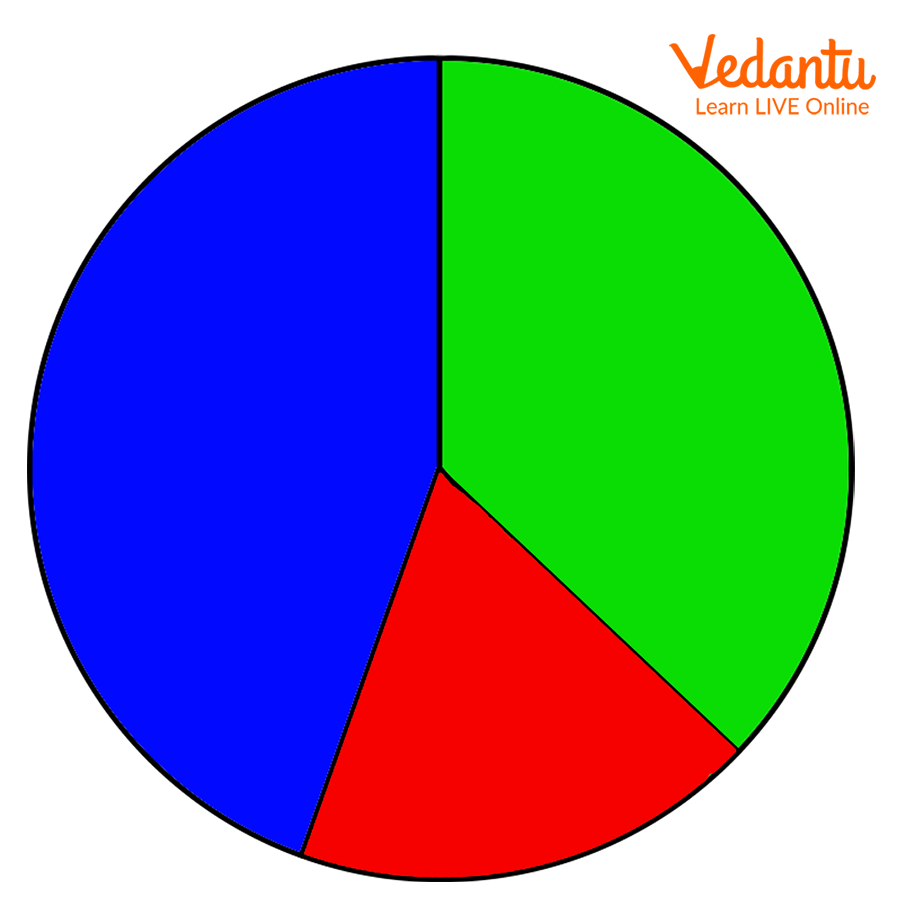Courses
Courses for Kids
Free study material
Offline Centres
More

# Let’s Learn and Know What are Unequal PartsLast updated date: 02nd Dec 2023
Total views: 141k
Views today: 4.41kHave you tried to divide anything into parts? This can be to distribute something among your friends or to take one part out and leave the rest of the parts to have later. How do you prefer to divide these parts? The general answer is “equally”. However, any object or item can also be divided into parts that are not equal in shape, size, or any other proportion. Not always do we get to deal with equal portions of an object or a number, so it is important that we know how unequal parts work. Let us learn more about this idea.

## Definition of Unequal Parts

When trying to divide a whole into two or more parts, if the parts do not match each other in proportion or any other aspect, the parts are said to be unequal parts. Let us consider a few examples to understand the concept better.

## Examples of Unequal Parts of a Whole

Let us consider the following image for understanding the idea of unequal parts clearly. The following image is a pie diagram that represents three different parts that a whole is divided into. The three parts are coloured green, blue, and red, respectively.A Pie Diagram Representing Three Unequal Parts Coloured Green, Blue, and Red, Respectively

From the given diagram, we can observe that the whole is divided into three parts that are not equal in proportion, that is, the green part is not equal to the blue part or the red part, and the blue part is not equal to the red part or the green part, and the red part is not equal to the green part or the blue part. So, these three parts can be called unequal parts of a whole.

Now, let us take this concept to a Mathematical level. Suppose there is a pie that needs to be divided into 5 equal parts. Then each portion of the pie will be represented in fractional notation as ⅕. However, if we were to divide the same pie into 5 unequal parts, then an option would be to divide it into the parts 1/15, 2/15, 3/15, 4/15, and 5/15. All these 5 parts are unequal and, when added together, give a whole. So, this was a Mathematical example of unequal parts.A Pie Divided into 5 Unequal Parts

## Conclusion

Unequal parts of an object or number are those parts that together form a whole but are not equal to each other in proportion of size, shape, or any other aspect. Equal parts are easy to deal with in Maths, but unequal parts are equally important and should not be left out. The concept should be understood properly to be able to solve problems based on unequal parts.

## FAQs on Let’s Learn and Know What are Unequal Parts

1. Why are unequal parts important in Maths?

Unequal parts are not something that can be avoided completely in Maths. There can be many problems that require us to deal with unequal parts, for instance, addition, subtraction, or other operations on fractions. Such problems require the knowledge of unequal parts.

2. How can unequal parts be distinguished from equal parts in fractions?

In equal parts, the reduced form of a fraction has the same numerators and denominators while in unequal parts, the numerators are not the same, even though the denominators might be the same.